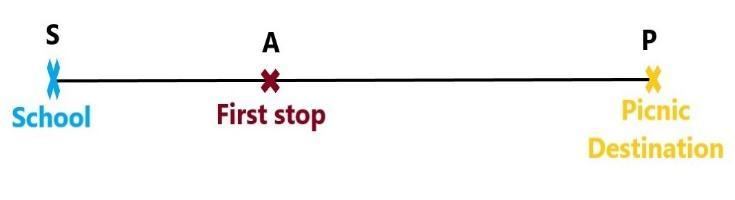QUESTION

# A picnic is being planned in a school for class VII. Girls are 60% of the total number of students and are 18 in number. The picnic site is 55 km from the school and the transport company is charging at a rate of Rs. 12 per km. The total cost of the refreshments will be Rs. 4280. Find:(a) The ratio of the number of girls to the number of boys in the class?(b) The cost per head if two teachers are also going with the class?(c) If their first stop is at place 22 km from the school, what percent of the total distance of 55 km is this? What percent of the distance is left to be covered?

Hint: For solving this question we will use the concept of percentage and make equations stepwise as per the data given in the question and then calculate the correct answer for each part.

Given:
Girls are 60% of the total number of students and are 18 in number.
First, we will find the total number of students in the class. Then we will find the number of boys and after that, we will determine the ratio of the number of girls to the number of boys.
Let us consider the total number of students is $N$ , the total number of girls is $G$ and the total number of boys is $B$ in the VII class. Then, we can write:
$N=G+B...........\left( 1 \right)$
Now, as $y\%$ of $N$ = $\dfrac{y}{100}\times N$ .
If $y=60$ then, 60% of $N=\dfrac{60}{100}\times N=0.6N$ .
It is given that there are 18 girls. Then,
\begin{align} & G=0.6N=18 \\ & \Rightarrow N=\dfrac{18}{0.6} \\ & \Rightarrow N=30...........\left( 2 \right) \\ \end{align}
Thus, the total number of students in the VII class is 30.

Now, we will find the total number of boys in the class using (1).
Putting, $N=30$ and $G=18$ in (1). Then,
\begin{align} & N=G+B \\ & \Rightarrow 30=18+B \\ & \Rightarrow B=30-18 \\ & \Rightarrow B=12 \\ \end{align}
Thus, there are a total of 12 boys in the class VII.
Then, the ratio of the number of girls to the number of boys $=\dfrac{G}{B}=\dfrac{18}{12}=\dfrac{3}{2}$.
Thus, the ratio of the number of girls to the number of boys is 3:2.

Calculation of the total cost of the picnic:
Let, $C$ represents the total cost of the picnic, ${{C}_{T}}$ represents the total transportation cost and ${{C}_{R}}$ represents the total cost of refreshment on the picnic.
Now, for calculating the total cost we will calculate total transportation cost and then add the refreshment cost in it.
It is given in the question that, distance of the picnic site from the school is $=55$ km.
Then, the amount charged by the transport company per km = Rs. 12.
For calculating the total cost of transportation we will multiply the total distance to be travelled and cost per km charged by the transport company. Then,
Total amount spent in availing the transport facility $={{C}_{T}}=55\times 12=$ Rs. 660.
It is given in the question that the total cost of refreshment is $={{C}_{R}}=$ Rs. 4280.
Then, the total cost of picnic $=C={{C}_{T}}+{{C}_{R}}=660+4280=$ Rs. 4940.

Now, for cost per head, we have to find the total number of persons going on the picnic and then divide the total cost by it to get the cost per head.
It is given in the question that the whole class is going on the picnic along with 2 teachers. So, for calculating the total number of persons going on the picnic we have to consider everyone.

Let, $P$ represents the total number of persons going on the picnic and $T$ represents the total number of teachers going on the picnic.
From (2) we have the total number of students in the VII class $=N=30$ .
It is given in the question that, the number of teachers going on the picnic $=T=2$ .
Then, the total number of persons going on the picnic $=P=T+N=30+2=32$ .
We have, total cost of picnic $=C=$ Rs. 4940.
Then, the cost per head $=\dfrac{C}{P}=\dfrac{4940}{32}=154.375$ .
Thus, the cost per head will be Rs. 154.375.
Now, it is given that their first stop is 22 km from the school. We can understand it easily with the help of the figure below:In the above figure, ‘S’ represents the school, ‘A’ represents the first stop from the school and ‘P’ represents the final picnic destination. Then,
We can write, SA = 22 km and SP = 55 km.
Percentage of SA in SP $=\dfrac{22}{55}\times 100=40%$ .
Thus, the distance of the first stop from school is 40% of the total distance.
After the first stop, the AP distance is left to be covered. Then,
\begin{align} & AP=SP-SA \\ & \Rightarrow AP=55-22 \\ & \Rightarrow AP=33 \\ \end{align}
Now, 33 km distance is left to be covered.
Percentage of AP in SP $=\dfrac{33}{55}\times 100=60\%$ .

Thus, after the first stop from school 60% of the total distance will be left to be covered.

Note: Here, a student should use the percentage concept accurately and proceed stepwise to find the answer for each part. Moreover, in the question, per head cost is asked so we have to add the number of teachers going on picnic also while calculating the per head cost.